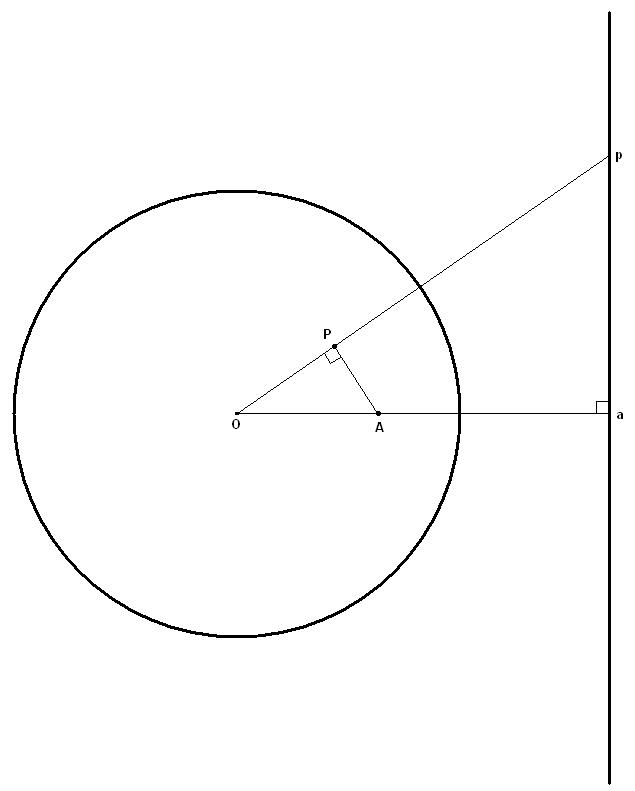#### You may also like### Symmetric Trace

Points off a rolling wheel make traces. What makes those traces have symmetry?### Mapping the Wandering Circle

In the diagram the point P can move to different places around the dotted circle. Each position P takes will fix a corresponding position for P'. As P moves around on that circle what will P' do?### Like a Circle in a Spiral

A cheap and simple toy with lots of mathematics. Can you interpret the images that are produced? Can you predict the pattern that will be produced using different wheels?

# The Line and Its Strange Pair

##### Age 14 to 16 Challenge Level:$a$ is the point on the line such that $Oa$ is perpendicular to the line, and A is its pair. For any point p on the line, it's pair $P$ forms a trianle $OAP$ which is similar to triangle $Oap$, since $$\frac{|OP|}{|OA|} = \frac{1/|Op|}{1/|Oa|} = \frac{|Oa|}{|Op|}$$. Therefore $\angle OPA$ is a right angle, and as p moves along the line, P traces out a cirlce with diameter $OA$.